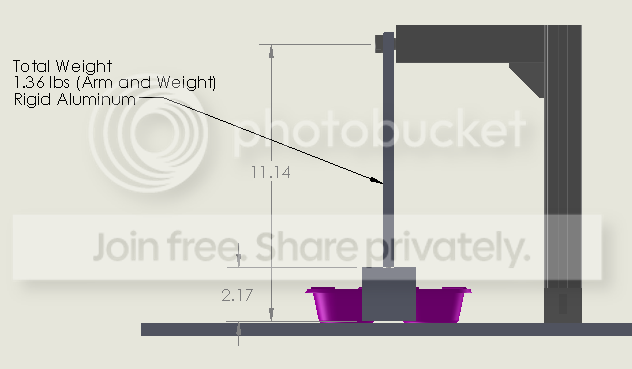User Name Remember Me? Password

 Kinematics and Dynamics Kinematics and Dynamics Physics Help ForumJul 12th 2017, 10:04 AM #1 Junior Member   Join Date: Jul 2017 Posts: 2 I need help with a Rigid Pendulum Calculation please I have a rigid body pendulum that is used to strike an object at the bottom of its swing. Ultimately I would like to know how much forced is the object being struck with. Could I do this with just knowing the velocity of the weight? I was thinking then I could get P=MV. Can someone please help e figure this out. Please see the attached diagrams.Jul 12th 2017, 02:25 PM #2 Senior Member   Join Date: Aug 2010 Posts: 389 You can calculate the velocity, momentum, and kinetic energy of the pendflulum when it hits the object but that will not tell you what force it hits the object with. That depends on other things, such as the elasticity of the pendulum head and the object, that are not given. If the pendulum is raised through angle $\displaystyle \theta$, then it is raised a height $\displaystyle h= L cos(\theta)$ where L is the length of the pendulum. Its potential energy there, relative to the bottom of the pendulum, is $\displaystyle mgh= mgL cos(\theta)$. The kinetic energy is 0. At the bottom the potential energy is 0 so the kinetic energy is [tex](1/2)mv^2= mgL cos(\theta)[tex] so $\displaystyle m= \sqrt{2gL cos(\theta)$. Last edited by HallsofIvy; Jul 12th 2017 at 02:33 PM.Jul 12th 2017, 03:55 PM #3 Junior Member   Join Date: Jul 2017 Posts: 2 I did something like this V=sqrt(2*g*L(1-cosθ) F= m*(ΔV/ΔT) The biggest factor there is T because. I used video of the collision and have a decent estimation of .025s for T. Thanks for your help!Jul 12th 2017, 05:01 PM #4 Senior Member   Join Date: Aug 2008 Posts: 113 I believe you're going to have to use rotational energy since the rod is rigid and is part of a two mass system, rod & end mass ... you'll also need to determine the pendulum's center of mass. If $d$ is the center of mass position relative to the pivot ... $M_p g d(1- \cos{\theta}) = \dfrac{1}{2} I_p \omega^2 \implies \omega = \sqrt{\dfrac{2M_p g d(1- \cos{\theta})}{I_p}}$ ... where $I_p = I_{rod} + I_{endmass}$ impact velocity = $L \omega$Tags calculation, pendulum, rigidThread ToolsShow Printable VersionEmail this Page Display ModesLinear ModeSwitch to Hybrid ModeSwitch to Threaded ModeSimilar Physics Forum Discussions Thread Thread Starter Forum Replies Last Post Matrixmake Theoretical Physics 0 Dec 15th 2012 11:03 PM Seesi Advanced Mechanics 1 Dec 4th 2012 07:53 AM madmartigano Kinematics and Dynamics 2 May 8th 2009 11:26 PM madmartigano Equilibrium and Elasticity 1 Apr 29th 2009 04:06 PM transgalactic Advanced Mechanics 17 Jan 30th 2009 09:02 AM